# Bread

5 of the same bread has the same weight as three bread and 4 kg of fruit. What weight has one bread?

x =  2 kg

### Step-by-step explanation:

5x = 3x + 4

5•x = 3•x + 4

2x = 4

x = 2

Our simple equation calculator calculates it.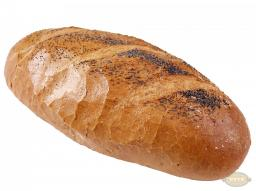Did you find an error or inaccuracy? Feel free to write us. Thank you!Tips to related online calculators
Do you have a linear equation or system of equations and looking for its solution? Or do you have a quadratic equation?

## Related math problems and questions:

• Birthday party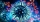For her youngest son's birthday party, the mother bought 6 3/4 kg of hotdog and 5 1/3 dozens bread rolls. Hotdogs cost 160 per kilogram, and a dozen bread rolls cost 25. How much did she spend in all?
• The fruit basketThe fruit basket is five times heavier than the empty basket. Filled is 20kg heavier than empty. Determine the weight of the fruit in the basket.
• Barrel 3Barrel with water weights 118 kg. When we get off 75% of water, it weights 35 kg. How many kg has an empty barrel?
• HomeworkMother and Kate together weigh 24.5 kg more than the father. Father weighs 7.6 kg more than mother. Father weight 44.8 kg more than Kate. Determine weights of father mother and Kate.
• Children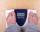Margaret and Zdena weigh the same and Petra 3 kg more. Together weigh 156 kg. How much do they weigh?
• Bob and Bobek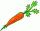Bobek has 2 cabbages and 5 carrots, Bob has 2 cabbages and 9 carrots. When Bobek stepped on the scale weight 5800 g and when Bob weight 8960 g. Actual Bob weight is 8 kg and Bobek 5 kg. How much weighs one carrot?
• Container with water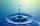The weight of a container with water is 2.48 kg. If the cast 75% water, the container with water has a weight of 0.98 kg. Determine the weight of the empty container. How much water was originally in the container?
• Cuboid 5Calculate the mass of the cuboid with dimensions of 12 cm; 0.8 dm and 100 mm made from spruce wood (density = 550 kg/m3).
• Balance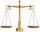The rod are 1.9 m long hanging weights 4 kg and 1 kg on ends. Where are centre of rod (distance from weight 4 kg) to be in balance?
• Water wessel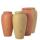The vessel containing water has a weight 19 kg. After pouring half the water its weigth 11 kg. What is the weight of the empty vessel?
• Can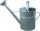Watering can full of water weighs 10 kg. Half-full can weighs 5.5 kg. How much weigh can?
• Stew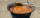To prepare stew for 10 loggers we need: 2.5 kg of potatoes, 0.8 kg of meat, 18 dag of flour, 30 dag onion and 1 bread. What is the required amount of raw materials for 50 participants camps where loggers eats 1.5 times more than a participant of the camp?
• Fruit importsCarriers import 18,324 kg of oranges from Spain. It was 16,857 kg less than mandarins and 2,645 kg more than bananas. a) how many kg did they bring mandarins? b) how many kg did bananas bring? c) how much total fruit?
• Volunteers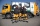Volunteers helped clean up 8.2 kg of trash in one neighborhood and 11 1/2 kg in another. They sent 2 3/5 kg to be recycled and threw the rest away. How many kilograms of trash did they throw away?
• Center of gravityThe mass points are distributed in space as follows - specify by coordinates and weight. Find the center of gravity of the mass points system: A1 [1; -20; 3] m1 = 46 kg A2 [-20; 2; 9] m2 = 81 kg A3 [9
• SandThe maximum weight of the car is 5000 kg. 10 m3 of sand must be transferred. How many times does a car have to go? (density of sand is 1500 kg/m3)
• Brick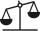Isosceles scale has on one side all brick and second weight 1 kg and 1/4 of brick. The balance is in equilibrium. What is the weight of a brick?